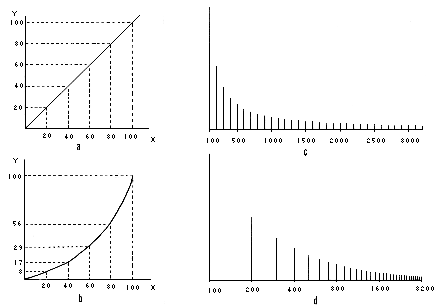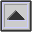LINEAR or LINEARITY
 Acoustics / Psychoacoustics / Electroacoustics

The RESPONSE of a system is linear when the output is directly proportional to the input, that is, any change in the input produces a proportional change in the output. When plotted on a graph, a straight line results.

A non-linear system is one where such a proportional relationship between input and output does not hold, as shown in the corresponding graph.Linear and non-linear relationships (a, b). Graphs (c, d) show a HARMONIC SERIES spectrum with two different scales on the horizontal axis. With (c) the frequency axis is linear, whereas with (d) the scale is logarithmic, with the result that octave intervals are equidistant and the harmonics are spaced increasingly closer. See: BASILAR MEMBRANE.

The result of the non-linear characteristics of the ear is the addition of harmonics when the incoming sound is of sufficient intensity.

Frequency, for instance, although it is a continuously variable PARAMETER, is not linear with respect to PITCH, since equal changes in frequency do not result in equal pitch changes. Instead, frequency must be doubled to get equal pitch changes, and therefore the relation may be called logarithmic (see MEL; for loudness, see DECIBEL, PHON, SONE).

With audio signals, linearity in their amplification is desirable, but when the signal has a large DYNAMIC RANGE, a LIMITER must be used to prevent overload and distortion, and in other cases, non-linear amplification called COMPRESSION must be used to reduce the signal's dynamic range.

In SOUND SYNTHESIS, non-linear techniques such as MODULATION are used to produce rich spectra from simpler sources.

home